# 神经网络中的反向传播算法算例2018年3月30日16:22:45

1 1827字阅读6分5秒

## 系数初始化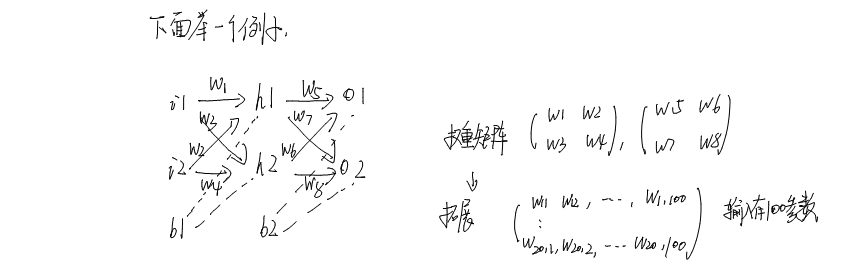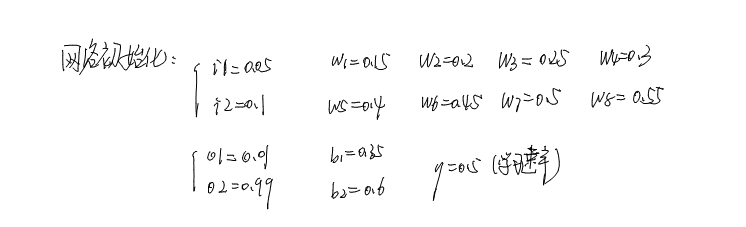1. w1 = 0.15;
2. w2 = 0.2;
3. w3 = 0.25;
4. w4 = 0.3;
5. w5 = 0.4;
6. w6 = 0.45;
7. w7 = 0.5;
8. w8 = 0.55;
9. i1 = 0.05;
10. i2 = 0.1;
11. b1 = 0.35;
12. b2 = 0.6;
13. o1 = 0.01;
14. o2 = 0.99;

## 前向传播算法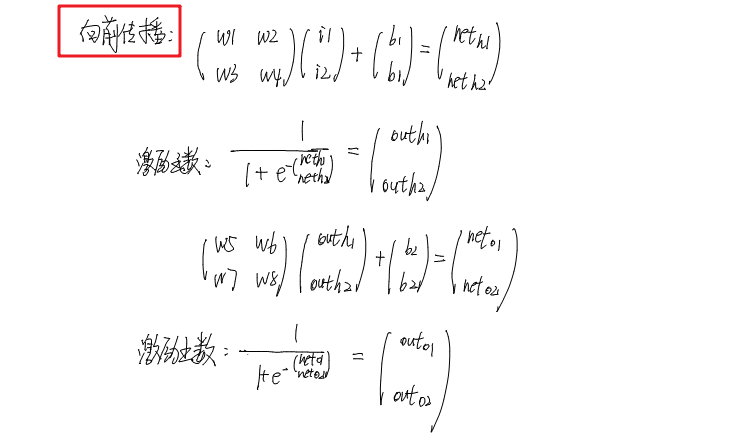1. {{netH1}, {netH2}} = {{w1, w2}, {w3, w4}}.{{i1}, {i2}} + {{b1}, {b1}}
2. >> {{0.3775}, {0.3925}}
3. {{outH1}, {outH2}} = 1/(1 + E^(-({{netH1}, {netH2}})))
4. >> {{0.59327}, {0.596884}}
5. {{netO1}, {netO2}} = {{w5, w6}, {w7,  w8}}.{{outH1}, {outH2}} + {{b2}, {b2}}
6. >> {{1.10591}, {1.22492}}
7. {{outO1}, {outO2}} = 1/(1 + E^(-({{netO1}, {netO2}})))
8. >> {{0.751365}, {0.772928}}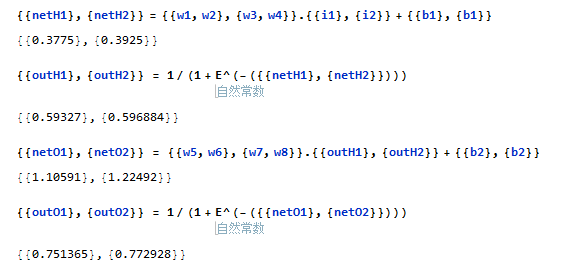## 反向传播算法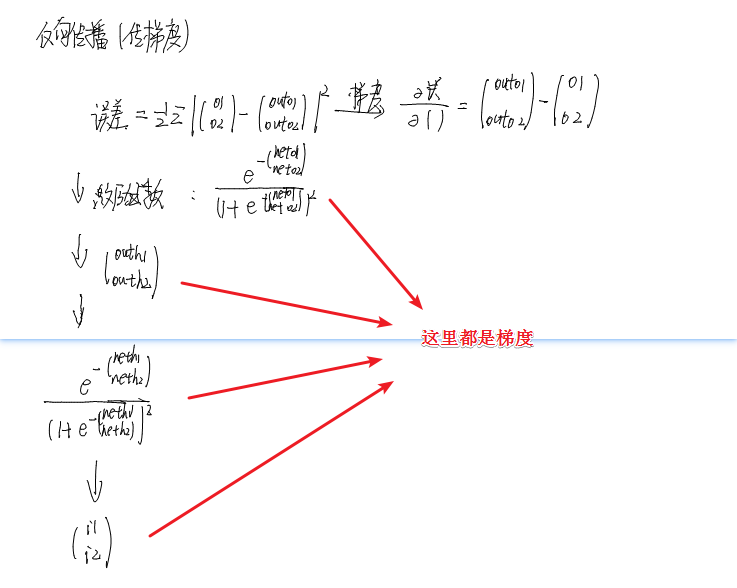1. dx1 = {{outO1}, {outO2}} - {{o1}, {o2}}
2. >> {{0.741365}, {-0.217072}}
3. dx2 = (E^(-({{netO1}, {netO2}})))/(1 + E^-({{netO1}, {netO2}}))^2
4. >> {{0.186816}, {0.17551}}
5. dx3 = {{outH1}, {outH2}}
6. >> {{0.59327}, {0.596884}}
7. dx4 = (E^(-({{netH1}, {netH2}})))/(1 + E^-({{netH1}, {netH2}}))^2
8. >> {{0.241301}, {0.240613}}
9. dx5 = {{i1}, {i2}}
10. >> {{0.05}, {0.1}}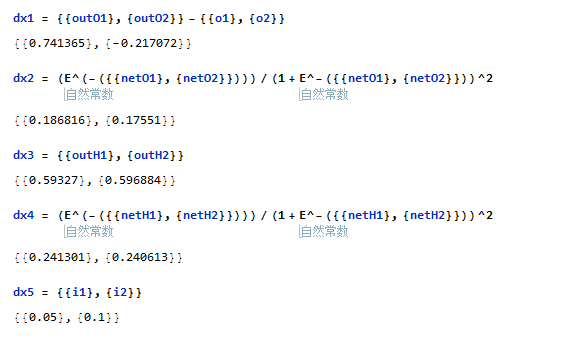## 参数更新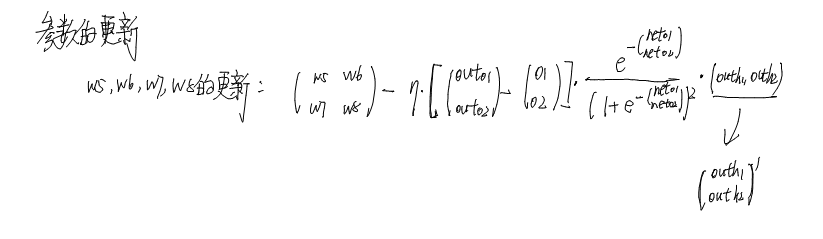1. {{w5, w6}, {w7, w8}} - 0.5*(dx1*dx2).Transpose[dx3]
2. >> {{0.358916, 0.408666}, {0.511301, 0.56137}}

1. {{w1, w2}, {w3, w4}} -  0.5*( Transpose[{{w5, w6}, {w7, w8}}].(dx1*dx2)*dx4).Transpose[dx5]
2.  >> {{0.149781, 0.199561}, {0.249751, 0.299502}}

## 完整过程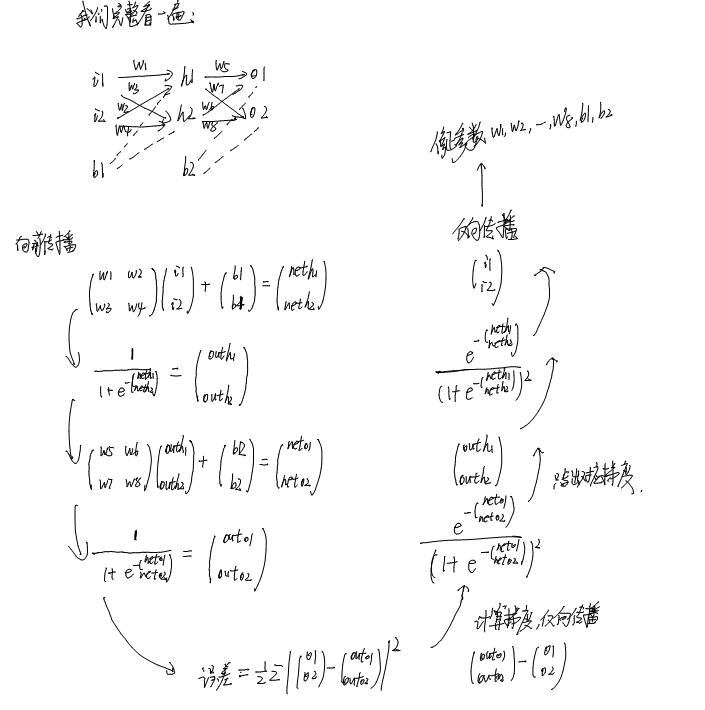## 一些话

• 微信公众号
• 关注微信公众号
•• QQ群
• 我们的QQ群号
•• 本文由 发表于 2018年3月30日16:22:45
• 转载请务必保留本文链接：https://mathpretty.com/9094.html